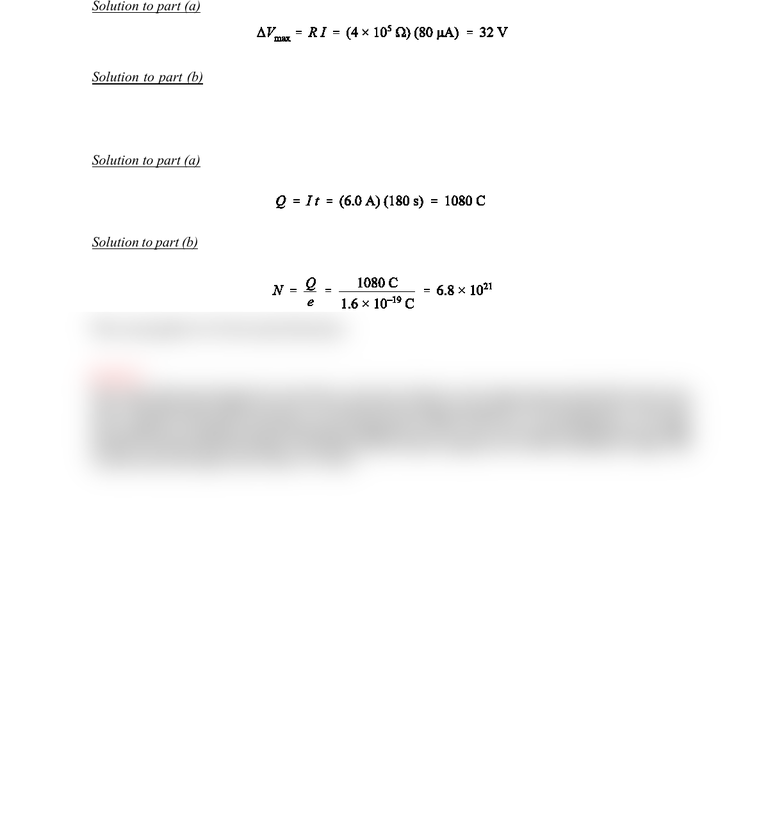# Physics 1028 Chapter Notes - Chapter 19: Molar Mass, Electrolytic Cell

2 views2 pages
School
Western University
Department
Physics
Course
Physics 1028
ProfessorSelection of Even–Numbered Problem Solutions
Chapter 19
P–19.8
Solution to part (a): We find with Ohm’s law:
(1)
Solution to part (b): For the lower resistance of wet skin we find accordingly Vmax = 0.16 V. Thus, the
danger of shock is much greater when a person’s skin is wet.
P–19.10
Solution to part (a): The charge passing through the cross–sectional area follows from the definition of the
current:
(2)
Solution to part (b): The number of electrons, which this amount of charge represents, follows from dividing
the total charge by the charge per electron, which is the elementary charge e:
(3)
This corresponds to 1% of a mol of electrons.
P–19.12
The current flowing through the electrolytic cell may be found as the charge deposited divided by the time
taken. Therefore this problem requires us to find the total charge deposited in 3.25 g of gold ions. The molar
mass of gold is 197 g/mol so the total number of gold ions is 9.94 × 1021 ions. Since the gold ions are singly
ionized, we obtain the total charge as the product of the number of gold ions with the elementary charge. The
current in the electrolyte cell is then I = 0.16 A.
Unlock document

This preview shows half of the first page of the document.
Unlock all 2 pages and 3 million more documents.

## Document Summary

Solution to part (a): we find with ohm"s law: (1) Solution to part (b): for the lower resistance of wet skin we find accordingly vmax = 0. 16 v. thus, the danger of shock is much greater when a person"s skin is wet. Solution to part (a): the charge passing through the cross sectional area follows from the definition of the current: (2) Solution to part (b): the number of electrons, which this amount of charge represents, follows from dividing the total charge by the charge per electron, which is the elementary charge e: (3) This corresponds to 1% of a mol of electrons. The current flowing through the electrolytic cell may be found as the charge deposited divided by the time taken. Therefore this problem requires us to find the total charge deposited in 3. 25 g of gold ions. The molar mass of gold is 197 g/mol so the total number of gold ions is 9. 94 1021 ions.